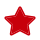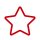Help Center> CloudTest> Best Practices> Typical Test Design Techniques
Updated on 2022-09-29 GMT+08:00

# Typical Test Design Techniques

Test design plays an important role in test activities. Test objects, scenarios, types, and environments are analyzed based on the test plan. Proper test techniques are selected based on the test policy to design test cases. Test design techniques include scenario analysis, equivalence partitioning, boundary value analysis, cause and effect graphing, decision table testing, orthogonal array testing, and more. Flexible use of test design techniques can help reduce redundant test cases, improve test coverage, maintainability, and reuse of test cases, reduce invalid test execution workload, and improve test effects.

#### Equivalence Partitioning

Equivalence partitioning is a testing technique that divides the input data of a system into equivalence classes of equivalent data. In each equivalence class, selecting all input data for testing is equivalent to selecting only one piece of input data for testing. If one piece of input data fails to detect any system error, all other input data in the equivalence class cannot detect any system error. Equivalence partitioning is used to select one piece of data from each equivalence class as the test input to improve the coverage of test scenarios and reduce invalid test workload.Equivalence partitioning includes valid equivalence classes and invalid equivalence classes. A valid equivalence class summarizes a set of reasonable and meaningful input data, that is, a regular path test input. It can be used to check whether the program implements the functions and performance specified in the specification. In contrast, an invalid equivalence class is a set of unreasonable or meaningless input data.

• Example 1: If the input condition is a Boolean value, a valid equivalence class and an invalid equivalence class can be obtained.

Input condition: whether to back up data.

Valid equivalence class: yes (TRUE).

Invalid equivalence class: no (FALSE).

• Example 2: If the input condition specifies the range of the input value, a valid equivalence class and an invalid equivalence class can be obtained.

Input condition: a number greater than 1 and less than 3.

Valid equivalence class: 2.

Invalid equivalence class: 0 and 4.

• Example 3: If the rules that the input data must comply with are specified, a valid equivalence class that complies with all rules and several invalid equivalence classes that violate the rules from different perspectives can be obtained.

Input condition: a positive integer.

Valid equivalence class: 1.

Invalid equivalence class: 0, –10, 10.1...

The equivalence partitioning technique focuses on the proper division of input values. When designing test cases, consider both types of equivalence classes. Ensure that not only reasonable data can be received, but also input in unexpected scenarios can be processed. When the equivalence partitioning technique is used for test design, list the possible inputs of the features to be analyzed, and divide and classify the equivalence classes. Each equivalence class after analysis is regarded as a test case. Tools such as tables or mind maps can be used to assist in equivalence partitioning. Equivalence partitioning is often used in conjunction with other techniques, such as boundary value analysis.

• For example, if the input data is months, which are integers ranging from 1 to 12, then 12 valid equivalence classes and one invalid equivalence class can be obtained, as shown in the following table.

Input Condition

Valid Equivalence Class

No.

Invalid Equivalence Class

No.

Month

1, 2, 3, 4, 5, 6, 7, 8, 9, 10, 11, 12

0001

13

1001

The following test cases can be created based on the equivalence classes.

No.

Test Case

Equivalence Class No.

0001

Input of a correct month

0001

0002

Input of an incorrect month

1001

#### Boundary Value Analysis

According to experience, a large number of errors occur on the boundary of the input or output range. Boundary value analysis is to select test data at and near the boundary of the divided equivalence class area and to design test cases. The following methods and principles can be followed:

• When the input condition specifies the value range

Select the boundary values and the values that are just beyond the boundaries as the test input. For example, if the input value is an integer ranging from 0 to 100, design test cases for values 0 and 100, and also for –1, 1, 99, and 101.

• When the input condition specifies the number of values

Select the maximum number, minimum number, minimum number – 1, and maximum number + 1 as the test input. For example, if the number of attachments to be uploaded ranges from 1 to 10, design test cases for values 1, 10, 0, and 11.

• When the input and output are ordered sets

Select the first and last elements of the set as the test input. For example, if the input is an ordered array and the array value ranges from 1 to 7, indicating Monday to Sunday, select 1 and 7 as the test input.

• If a group of values (n) of the input data are specified and the program needs to process each input value separately, n valid equivalence classes and one invalid equivalence class can be established.
• Analyze the specifications and find out other possible boundary conditions.After the preceding steps are performed, a large number of test items may be generated, which may be repeated and can be combined. Boundary value analysis is often used together with equivalence partitioning. Generally, boundary values are selected in equivalence classes.